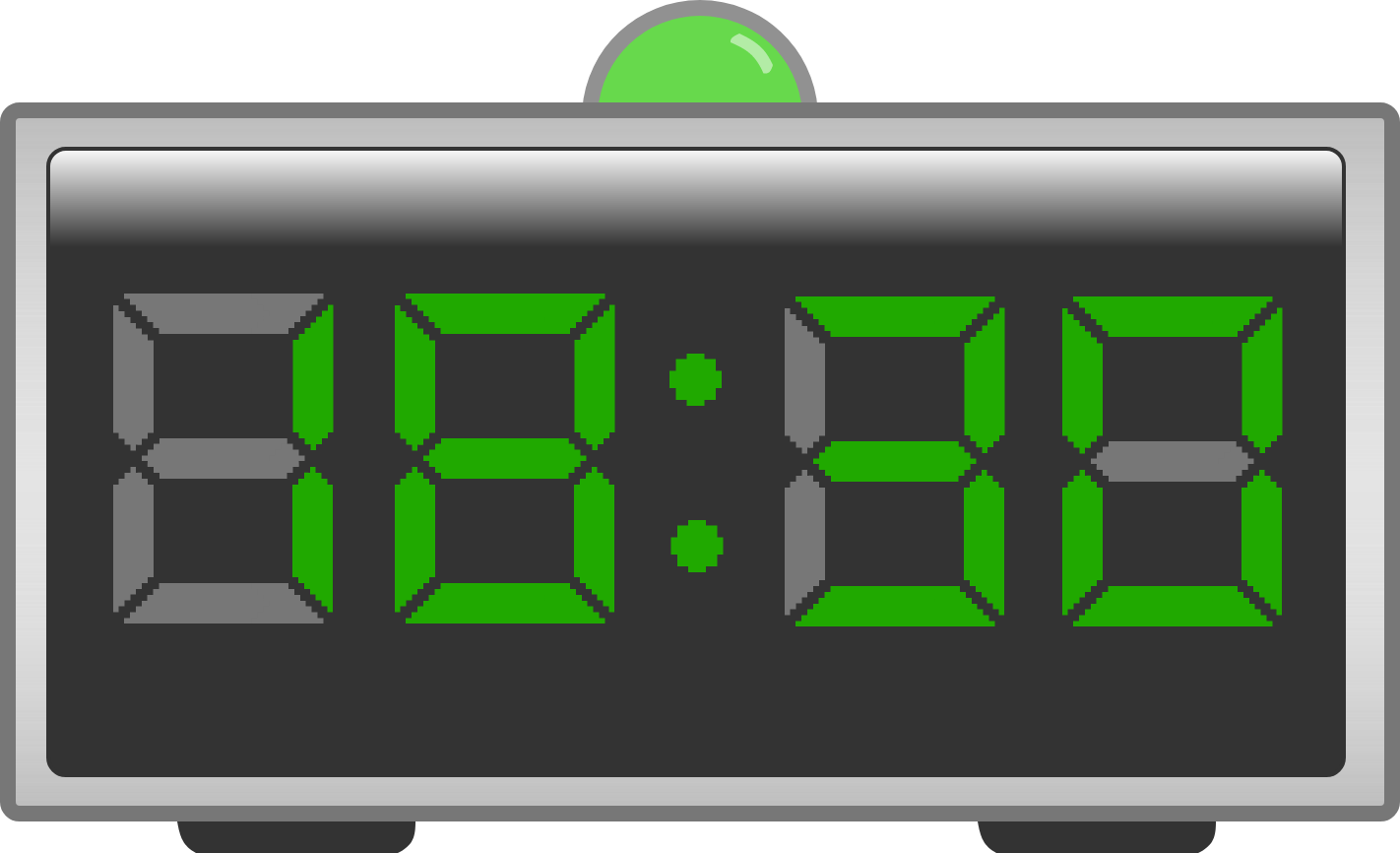# Mandelbrot's Clock

Calculus Level 3Mandelbrot has a $24$-hour digital clock with a light above it.

Every minute, a computer in the clock calculates a complex number $c = \big(\frac{1}{6}h - 2\big) + \big(\frac{1}{15}m - 2\big)i,$ where $h$ is the hour and $m$ is the minute. If the orbit of $z_n$ for $z_{n + 1} = z_n^2 + c$ with $z_0 = 0$ tends to infinity, the light turns green, but if the orbit is bounded, the light turns red.

For example, at $18$:$30$, $c = \big(\frac{1}{6}18 - 2\big) + \big(\frac{1}{15}30 - 2\big)i = 1,$ and this gives the sequence $0, 1, 2, 5, 26, \dots,$ which tends to infinity, so the light on the clock would be green. But at $6$:$30$, $c = \big(\frac{1}{6}6 - 2\big) + \big(\frac{1}{15}30 - 2\big)i = -1$, and this gives the sequence $0, -1, 0, -1, 0, \dots,$ which is bounded, so the light on the clock would be red.

If you were to look at the clock at a random time during the day, would the light above the clock be more likely to be green or red?

×